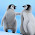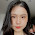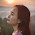## Saturday, June 4, 2016

### How to solve classification and regression fast, faster, and fastest

(post by Zeyuan Allen-Zhu)

I am often asked what is the best algorithm to solve SVM, to solve Lasso Regression, to solve Logistic Regression, etc. At the same time, a growing number of first-order methods have been recently proposed, making it hard to track down the state-of-the-arts. I feel it perhaps a good idea to have a blog post to answer all these questions properly and simultaneously.

Consider the general problem of empirical risk minimization (ERM):
\begin{equation}\label{eqn:primal}
\min_{x\in \mathbb{R}^d} \Big\{ F(x) := \frac{1}{n} \sum_{i=1}^n f_i (\langle a_i, x \rangle) + \psi(x) \Big\}\tag{1}\end{equation}
Here, each $a_i \in \mathbb{R}^d$ can be viewed as a feature vector, each $f_i(\cdot)$ is a unvariate loss function, and $\psi(x)$ can be viewed as regularizers such as the $\lambda \|x\|_1$ or $\frac{\lambda}{2}\|x\|_2^2$. There are naturally four classes of interesting classification or regression problems that fit into the above framework. Namely,
• Case 1: $f_i(x)$ is smooth and $F(x)$ is strongly convex. Example: ridge regression.
• Case 2: $f_i(x)$ is smooth and $F(x)$ is weakly convex. Example: Lasso regression.
• Case 3: $f_i(x)$ is non-smooth and $F(x)$ is strongly convex. Example: SVM.
• Case 4: $f_i(x)$ is non-smooth and $F(x)$ is weakly convex. Example: L1-SVM.
Somewhat surprisingly, it is not necessary to design an algorithm to solve each of the four cases above. For instance, one can "optimally" transform an algorithm solving Case 1 to algorithms solving Case 2,3 and 4 (link to paper). Since full gradient based methods are too slow for large-scale machine learning, in this post I'll summarize only stochastic methods.

[[edit remrak: one may also consider a few other interesting cases, including: Case 5, $f_i(x)$ is non-convex but $F(x)$ is strongly convex; Case 6, $f_i(x)$ is non-convex but $F(x)$ is weakly convex; Case 7, $f_i(x)$ is non-convex and $F(x)$ is non-convex too. Case 5 was first studied by Shai Shalev-Shwartz in this paper. I have slightly better results for Case 5 and 6 in this paper. As for Case 7, a recent progress is this paper.]]

## Running-Time Summaries

There are three classes of stochastic first-order methods, and the best known running times are respectively:
 Column 1: SGD Method (fast) Column 2: Non-Accelerated Methods (faster) Column 3: Accelerated Methods (fastest) Case 1 $O\Big(\frac{G d}{\sigma \varepsilon}\Big)$ $O\Big(\big(nd + \frac{L d}{\sigma}\big) \log\frac{1}{\varepsilon} \Big)$ $O\Big(\big(nd + \frac{\sqrt{n L} d}{\sigma}\big) \log\frac{1}{\varepsilon} \Big)$ Case 2 $O\Big(\frac{G d}{\varepsilon^2}\Big)$ $O\Big(nd \log\frac{1}{\varepsilon} + \frac{L d}{\varepsilon} \Big)$ $O\Big(nd \log\frac{1}{\varepsilon} + \frac{\sqrt{n L} d}{\sqrt{\varepsilon}} \Big)$ Case 3 $O\Big(\frac{G d}{\sigma \varepsilon}\Big)$ $O\Big(nd \log\frac{1}{\varepsilon} + \frac{G d}{\sigma \varepsilon} \Big)$ (useless) $O\Big(nd \log\frac{1}{\varepsilon} + \frac{\sqrt{n G} d}{\sqrt{\sigma \varepsilon}} \Big)$ Case 4 $O\Big(\frac{G d}{\varepsilon^2}\Big)$ $O\Big(nd \log\frac{1}{\varepsilon} + \frac{G d}{\varepsilon^2} \Big)$ (useless) $O\Big(nd \log\frac{1}{\varepsilon} + \frac{\sqrt{n G} d}{\varepsilon} \Big)$
In the above table, $L$ is the smoothness parameter for Cases 1/2, and (square root of) $G$ is the Lipschitz continuity parameter of $f_i$ for Cases 3/4, and $\sigma$ is the strong convexity parameter for Cases 1/3. It is clear from the table above that non-accelerated methods should not be used for cases 3 and 4, and also clear (using AM-GM inequality) that column 3 is faster than column 2.

If one simply wants to know how to obtain such running times, then the short answer is.
• SGD results are more-or-less folklore, see for instance Section 3 of Elad Hazan's text book.
• Non-accelerated results: Case 1 is first obtained by SAG to the best of my knowledge. Case 2 is first obtained by Yang and me (by shaving off a log factor from SVRG). Case 3/4 are not interesting but anyways obtainable for instance using this reduction. In practice, SVRG and SVRG++ indeed outperform SGD for Cases 1 and 2 based on my experience.
• Accelerated results: The tightest results for Cases 1/2/3/4 are first obtained by the new method Katyusha. If one is willing to lose a few log factors, these cases were first obtained by AccSDCA in 2013. Based on my experience, at least for Cases 1+2, Katyusha seems to give the best practical performance. For Cases 3+4, I would suggest APCG (see later).
If one is interested in, from a high level, how such results are obtained, I categorize all existing methods into dual-only methods, primal-only methods, and primal-dual methods.

## Dual-Only Methods (SDCA, APCG, etc.)

Due to technical reasons, it is easier (and somehow earlier in history) to study ERM problems from the dual perspective. The dual problem of \eqref{eqn:primal}:
\begin{equation}\label{eqn:dual} \min_{y \in \mathbb{R}^n} \Big\{ D(y) := \frac{1}{n}\sum_{i=1}^n f_i^*(y_i) + r^*\Big(-\frac{1}{n} \sum_{i=1}^n y_i a_i \Big) \Big\}
\tag{2}
\end{equation}
Above, $f_i^*$ and $r^*$ are respectively the so-called Fenchel dual of $f_i$ and $r$ respectively. For starters, Fenchel duals are easily computable for most applications; as a concrete example, if $r(x) = \frac{\lambda}{2}\|x\|_2^2$ is the L2 regularizer, then $r^*(x) = \frac{1}{2\lambda} \|x\|_2^2$. Section 5 of this paper provides lots of examples.

Note that the dual objective $D(y)$ is undefined for Cases 2 and 4. Although $D(y)$ is defined for Case 3, it is in theory impossible to translate an approximate dual solution $y$ to a primal solution. For such reasons, in theory people directly analyze how to minimize $D(y)$ for Case 1, and remember, such an algorithm can be turned into solvers for Cases 2/3/4 through reduction.
One can prove that if $F(x)$ is $\sigma$-strongly convex, then $D(y)$ is $(1/n + 1/sigma n^2)$-smooth with respect to each coordinate. For this reason, one can apply coordinate descent to minimize $D(y)$ directly, which results in the SDCA method; or apply accelerated coordinate descent to minimize $D(y)$ directly, which results in the APCG method. Note that SDCA is a non-accelerated method (column 2 of the table) and APCG is an accelerated method (column 3).

Although (accelerated or not) coordinate descent was well-known in optimization, it is not immediately clear how to apply them to minimize $D(y)$ in \eqref{eqn:dual} at a first glance, mainly due to the existence of the Fenchel conjugates. The APCG paper provides a nice summary for beginners.

I have another blog post discussing coordinate descent methods in details.

## Primal-Only Methods (SGD, SVRG, etc.)

A stochastic method is call primal if it directly computes $f_i'(\cdot)$ for one random sample $i$, and update $x$ accordingly. Primal-only methods are more desirable for lots of reasons. First, one primal methods avoid the accuracy loss when turning from dual variables to primal. Second, one can avoid the potentially involved Fenchel computation. Third, consider the more general problem of \eqref{eqn:primal} by replacing each $f_i(\langle a_i, x\rangle)$ with the more general form $f_i(x)$, this problem can only be solved by primal-only methods because its dual (in some sense) does not exist.

The first stochastic method is stochastic gradient descent (SGD). Ignoring the existence of the regularizer $\psi(x)$, the SGD method iteratively updates $x_{k+1} \gets x_k - \eta \cdot f_i' (\langle a_i, x\rangle) a_i$ where $\eta$ is some learning rate and $i\in [n]$ is a random sample. It is clear from this formulation that SGD replaces the full gradient $\nabla := \frac{1}{n} \sum_{i=1}^n f_i' (\langle a_i, x\rangle) \cdot a_i$ with an unbiased random sample $\tilde{\nabla} := f_i' (\langle a_i, x\rangle) \cdot a_i$, which is $n$ times faster to compute.

The first theoretical breakthrough on primal-only methods is SAG (to the best of my knowledge), which introduces the variance-reduction technique in order to match the running time of SDCA (and thus column 2 of the table). Approximately a year later, SVRG and SAGA are introduced to replace SAG with not only a simpler proof, but much better practical performance. The key idea of these methods is to design a better $\tilde{\nabla}$ so that its expectation $\mathbb{E}[\tilde{\nabla}]$ stills equals to the full gradient $\nabla$, but somehow approaching to zero. Unfortunately, all these variance-reduction based methods only match the non-accelerated running time (column 2).

In this March 2016, I finally obtained the first accelerated method (thus column 3) that is primal-only. The key idea there is to introduce a momentum plus a negative momentum on top of variance-reduced $\tilde{\nabla}$. Interested readers can find my method here, and I plan to give a more detailed survey on variance-reduction based methods later this summer.

## Primal-Dual Methods (SPDC)

One can also solve \eqref{eqn:primal} from a saddle-point perspective. Consider
$$\min_{x\in \mathbb{R}^d} \max_{y \in \mathbb{R}^n} \Big\{ \phi(x,y) := \frac{1}{n} y^T A x + \psi(x) - \frac{1}{n}\sum_{i=1}^n f_i^*(y_i) \Big\}$$
where $A = [a_1,\dots,a_n]^T \in \mathbb{R}^{n\times d}$ is the data matrix. One can prove that $F(x) = \max_y \phi(x,y)$ and $D(y) = - \min_x \phi(x,y)$, and therefore to solve the original ERM problem it suffices to solve this saddle-point problem.

In 2014, Zhang and Xiao provided an accelerated, stochastic method SPDC (column 3) that directly solves this saddle-point problem. It is built on the so-called accelerated primal-dual methods of Chambolle and Pock, which I also plan to write a blog post about it some time in the future. Unfortunately, I know people who report to me that the parameter tuning steps for SPDC may be too complicated in practice, but I haven't tried it myself so I can't say for sure.

## Final Remarks

In the above summary I have injected lots of my own (and perhaps controversial) opinions into the discussions above. For instance, one can also regard SDCA as a primal-dual methods because it somehow "also maintains primal variables". At the same time, the running times provided in my table are tight and the (almost) matching lower bounds recently shown by Woodworth and Srebro. Finally, let me conclude with a performance plot comparing Katyusha to other state-of-the-arts:1.This comment has been removed by the author.

2."If you have option to avoid subgradient methods - it will be really good idea" - EE364B, https://youtu.be/B51GgGCHBRk?t=1981

FYI other fun moments from S.Boyd class: https://sites.google.com/site/burlachenkok/ee364b

All algorithms which you describe Hessian has special structure - so there is no reason at Earth use any first-orde method to them.

3.The people in ML called SGD something which is called Stochastic Subgradient method in Convex Optimization....Even the simplest algorithm with fixed step length (learning rate) it diverges or not converge...If you have correct weighting (not summable, but square summable) then algorithm converge, but it take a long time....

In any case I have own implementation of several calssical ML algorithms which are reduced to convex optimization I will be glad to compare my algorithm with others. Let me know if know place where I can try it.

4.This comment has been removed by the author.

5.I returned home today and plugged my iPad into my laptop and while iTunes did not offer to remove repair corrupted files the passcode I was able to access the raw filesystem using iFunBox, which I could not do when my iPad was connected to any of my other computers.

6.Fantastic blog.Really thank you! I truly feel this amazing site requirements far more consideration.Fantastic.This is one wonderful blog article.Much thanks again
shopify app free shipping bar, Shopify free shipping bar app, best autoketing

7.Wow I can say that this is another great article as expected of this blog.I recently came across your blog and have been reading along. I am hoping the same best work from you in the future as well.
sales pop master free sales notifications, autoketing app

8.I don't know what to say except that I have enjoyed reading I will keep visiting this blog very often.Waiting For More new Update and I Already Read your Recent Post its Great Thanks.

9.I feel good when reading your posts. Those are what I want to know. Thank you for introducing useful information to us
autoketing
email with love by autoketing

10.11.I really appreciate for this great information,It is very nice of you to share your understanding. Thank you so much. I usually wait for your pieces of writing.
abcya3 for school jogos motox3m3 gogy for school

12.Free Crime city 3d 2 games
Games Flyordieio
The story of cinderella Games unblocked
I recently found many useful information in your website especially this blog page. Among the lots of comments on your articles. Thanks for sharing.

13.Hope you can write more posts. I like them very much. To me, they really help me sometimes. Thanks for sharing your knowledge.
free game Battle for the Galaxy Break The Cup For Free online game Rapunzel Fashionista Busy Day for girl

14.I really want to express my gratitude to you. The articles bring insight and knowledge.Keep your work.I think many people love and need them.
Ride The Bus Simulator online free game Talking Tom Gold Run Online game for free best game Tomb Runner

15.Great post. and great website. Thanks for the information! This is my first time i visit here and I found so many interesting stuff in your blog especially it's discussion. thank you.
Xmas magic tiles online game
Truck physics games for girls
Ice cream maker game online

16.What a blog post!! Very informative and also easy to understand. Looking for more such comments!! Do you have a facebook? I recommended it on digg. The only thing that it’s missing is a bit of new design.
Elsa breakup drama best online game
Fire truck crazy race games for boy
Unblock the ball games online